# The proper way to dynamically create a two-dimensional array in C

When I began working with matrices in C, I was obsessed with malloc. I almost never defined static arrays, because I thought that malloc turned me into a ‘Memory God’ capable of managing memory efficiently and improving loading and response times of my application, all while not putting the burden on the operating system. So, I quickly figured out how to dynamically allocate a two dimensional array. This is what I used to do in order to create a 10×5 array of integers:

After the job was done, I used to free the matrix like this:

Someone might ask: “What’s wrong with that?”. Well, for most intents and purposes this code works perfectly fine, but there is one subtle issue with this code and chances are that, if you use something like that, you are not even aware of it. The problem with this code is that it goes against the way C allocates static matrices in memory.

When you create a static 3×3 matrix in C like this:

```int staticMatrix;
```

the memory blocks allocated for each column stand each next to each other as demonstrated below: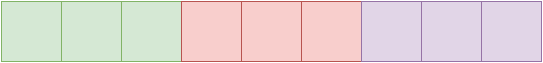The green blocks are the columns of the first row, the red blocks are the columns of the second row and the purple blocks are the columns of the third row. This two-dimensional array or array of arrays is actually a contiguous block in memory.

This is also the reason why the second dimension is always required when passing a 2D array to a function˙ the compiler needs to now the offset between rows, because in memory there are no actual dimensions. To better explain this, let’s assume you have this function that takes a 2D array and does some operations on it (we do not care about the exact operations):

```void workWithMatrix(int matrix[]);
```

We are required to specify the number of columns, but not the number of rows (although we can include that too, if we want). That’s because, every time we use the indexer operator, the compiler translates our statement into a pointer. If the following statement exists within workWithMatrix:

```int value = matrix;
```

The compiler will “translate it” to this:

```int value = *(matrix + 2*3 + 1);  //*(matrix + 7)
[\c]

If the statement was:

int value = matrix;
[\c]

It would translate into this:

int value = *(matrix + 1*3 + 1);  //*(matrix + 4)
```

Notice the number 3, that’s the offset, a.k.a. the number of columns, a.k.a. the number of blocks between two rows.

This is a visual illustration of how the compiler treats your 2D array as a single dimension one.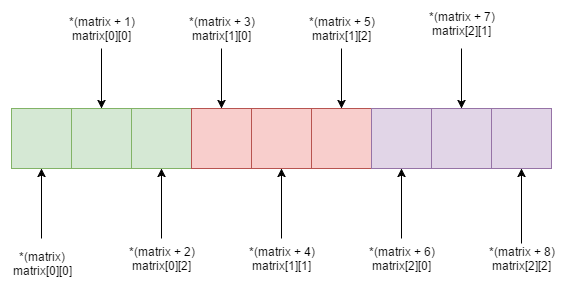The following is a typical illustration of how the dynamic matrix we created with malloc gets stored in memory: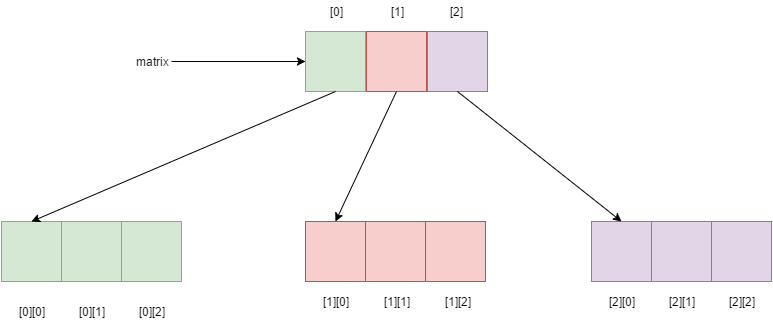As you can see, the blocks may be consecutive or they may not, there is no guarantee. That rarely causes a problem in most C programs, but when you have some advance logic, you want the matrix to be stored in contiguous blocks (for example, when creating a derived data type in MPI). To fix this, let’s introduce the correct way to dynamically allocate two dimensional arrays. What we want is to emulate a static array and from what we’ve seen, a statically defined 2D array is actually a one dimensional array (since there’s no such thing as a dimension in memory). So, when we want an NxM matrix, we first need to create a one dimensional array containing NxM elements, and then we create an array of pointers and make them point to the correct place in memory.

In the following example, N=3 and M=3.

In steps:

1. Create a one-dimensional array of 3×3=9 elements: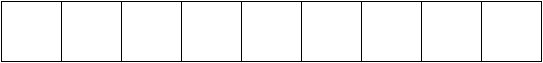2. Create a one-dimensional array of N=3 pointers (equal to the number of rows):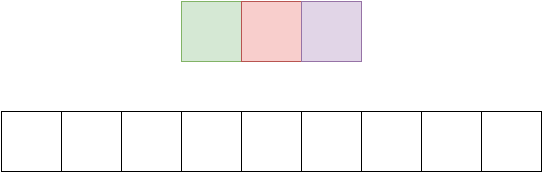3. Loop through the array of pointers and make them point to the start of each row, first one to array, second one to array and the third one to array: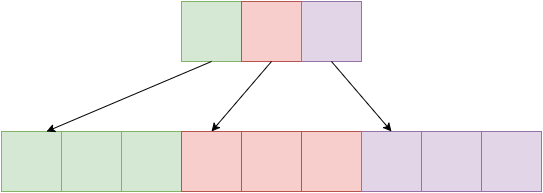4. Use the array of pointers (which is an int** for integers, a float** for floats, etc.) to reference the elements using the indexer operator []

And the long awaited code to achieve the above:

After we’re done with the matrix, we need to free any memory associated with it. To do that, we need to use a different approach than just looping through the rows and freeing the columns. This approach is actually faster and simpler. Straight to the code:

Remember that matrix is an array in and of itself. So we just need to free that, and also free the array of pointers. Pretty simple stuff.

I’ve also included some more helper functions for matrix operations. You can check them out here

I hope you liked this post and found it helpful. Have a nice day!

# The secret of C one-dimensional arrays

One thing that confuses newcomers to the C language, whο usually are newcomers to programming altogether, is the distinction between pointers and arrays. When you start learning C, you first learn about arrays. Every book or course teaches you statically allocated one dimensional arrays first. A statically allocated array is declared like this:

```int array; //Declares an array of 10 integers
```

One thing that confuses newcomers to the C language, whο usually are newcomers to programming altogether, is the distinction between pointers and arrays. When you start learning C, you first learn about arrays. Every book or course teaches you statically allocated one dimensional arrays first. A statically allocated array is declared like this:

```int* dynamicArray= malloc(8*sizeof(int));
```

Let’s dissect the above statement. We have a pointer to an integer (array). If you’ve studied pointers, you know very well that a pointer to an integer is actually an integer itself that contains the address of an integer in memory. What happens next is that we use the malloc function that allocates memory for 10 integers on another memory segment, the heap. A ‘stack vs heaps argument’ could have an article on its own, but for now let’s just focus on the key difference between the two, which is the fact that everything that’s put on the stack lives as long as the function that created it lives. When that functions’ job is done, it gets kicked out of the stack and so are any variables, arrays or structures that were defined within it. On the contrary, everything allocated on the heap lives as long as the whole program lives in memory, unless we explicitly free that part of the memory. That’s another essential part of dynamic arrays that we are usually taught˙ when we’re done with them we should ‘free’ them:

```free(dynamicArray);   //Any memory that was used for this array now belongs to the operating system to utilize
```

The first question that popped into my mind when I started learning that stuff was: “How the hell can I tell the difference between a pointer to a single integer and a dynamically allocated array of who-knows-how-many integers?”

The missing piece of the puzzle was a small bit of information about arrays:
The name of the array (a.k.a the variable) is a pointer to the first element of the array.

Sounds confusing? Let me explain. The variable that you use to handle the array, like ‘dynamicArray’ in the above example, is actually a pointer to the first element of the array. Let’s say that our dynamicArray contains the following integers: 6,4,5,7,9,1,0,2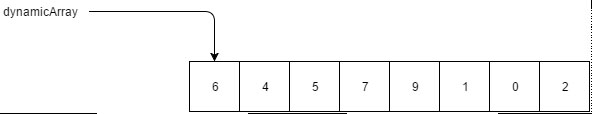So we can get the first element just by dereferencing the pointer:

```printf("First element = %d\n", (*dynamicArray));
```

Now, what about the second element? We can get that by moving the pointer one position to the right:

```printf("Second element = %d\n", *(dynamicArray + 1));
```

The third element by moving two positions to the right:

```printf("Third element = %d\n", *(dynamicArray + 2));
```

etc…

This sounds similar to the zero-based indexing of a statically declared array. If we wanted to get the first element of the array, we statically defined in the beginning of this article we would use this syntax:

```int firstElement = array;
```

When you first learn about the zero-based index everyone tells you “that’s the way it is” and you’re left wondering why this:

```int firstElement = array;
```

Is better than this:

```int firstElement = array;
```

Now you know the reason why. It’s because the variable name points to the first element. And guess what, this applies to statically defined arrays too!

You see, the ‘dereference method’ of accessing array elements is not exclusive to arrays created using malloc. You can use it in plain old statically defined arrays too! Because the ‘array’ variable we used above is also a pointer. To better explain this, I’ve put together a small console application that shows that you can use both the indexing operator ( [] ) and the pointer dereference operator ( * ) with any array, whether statically or dynamically defined:

We start by defining two identical one dimensional arrays, the first one on the stack and the second one on the heap. We then go on and print the first and fifth element of each array using both methods, therefore proving that both methods can be used regardless of the way the array has been defined.

#### How to pass arrays as arguments

Now that we’ve dug deeper in the world of one dimensional arrays, let’s see how we can pass them around functions. To do that, let’s write a ‘printArr’ function that will print the contents of a one-dimensional array.

For a function to print an array, it needs to know two things: the array (duh) and its size. So we need a function that will take two arguments and since it won’t return anything, it can be void. So we have the function’s signature!

```void printArr(int array[], int size);
```

The ‘[]’ syntax tells the compiler that this function expects its first argument to be an array.

Here’s the implementation. Nothing fancy, just a loop over the array contents and a call to printf

Now let’s use it to print the two arrays we defined earlier:

```printf("Array contents:\n");
printf(array, 8);

printf("dynamicArray contents:\n");
printArr(dynamicArray, 8);
```

The results are: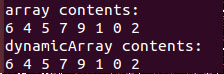Which is correct, of course.

There is also another way to pass an array to a function. Instead of using the [] syntax, we could just pass a pointer since every array is actually a pointer to the first element!There is also another way to pass an array to a function. Instead of using the [] syntax, we could just pass a pointer since every array is actually a pointer to the first element!

There is also another way to pass an array to a function. Instead of using the [] syntax, we could just pass a pointer since every array is actually a pointer to the first element!

```printf("Array contents (alternative method):\n");
altPrintArr(array, 8);

printf("dynamicArray contents (alternative method):\n");
altPrintArr(dynamicArray, 8);
```

#### What should I use

Okay, so, we learned that there are two ways to access an arrays’ content and there also are two ways to pass an array to a function. But which one should I use? Well, when it comes to accessing array elements, I personally believe that using the indexer operator makes your code cleaner, nicer and more comprehensible. As far as argument passing is concerned, someone might say that the pointer approach can get confusing when someone else reads your code because there is no immediate way of telling if the function accepts a pointer to a single element or an array.

It all comes down to personal preference though. I personally prefer using the indexing operator for accessing elements and I like using the pointer syntax for array arguments.

#### Conclusion

That was it! I hope you found this article helpful in your quest to master not only one-dimensional arrays, but C in general. The rest of the code can be found on GitHub. More stuff on C coming up soon. Thanks for reading. Keep coding!!